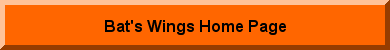Deviation and Interpolation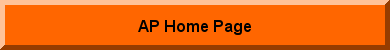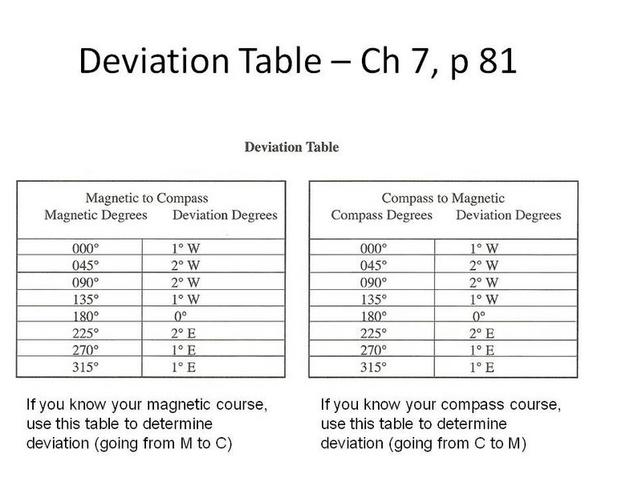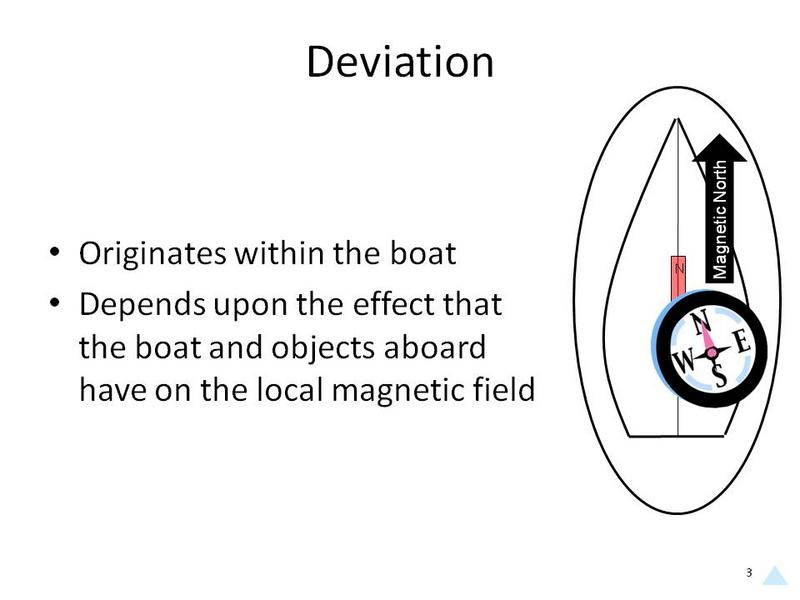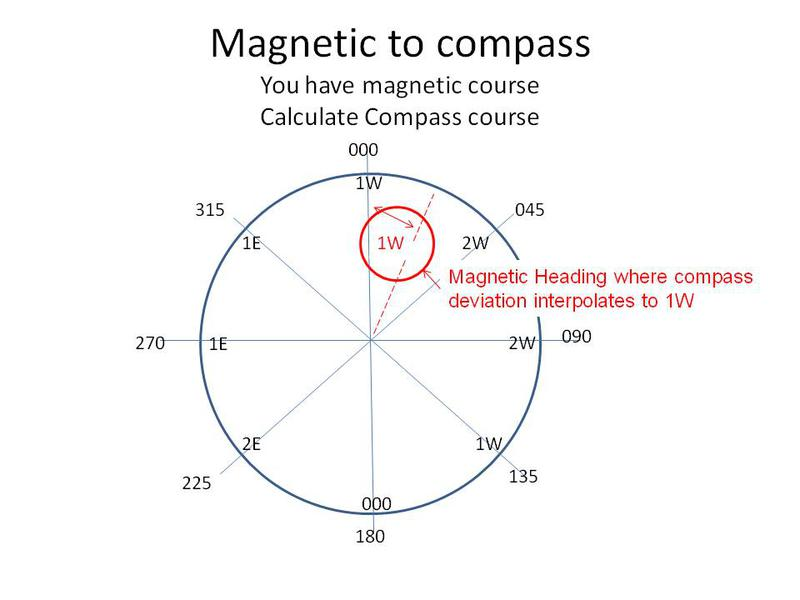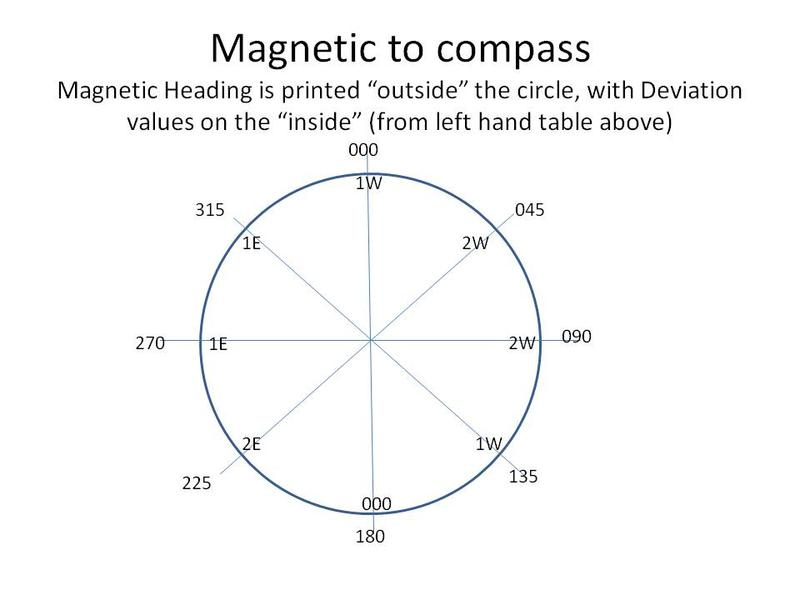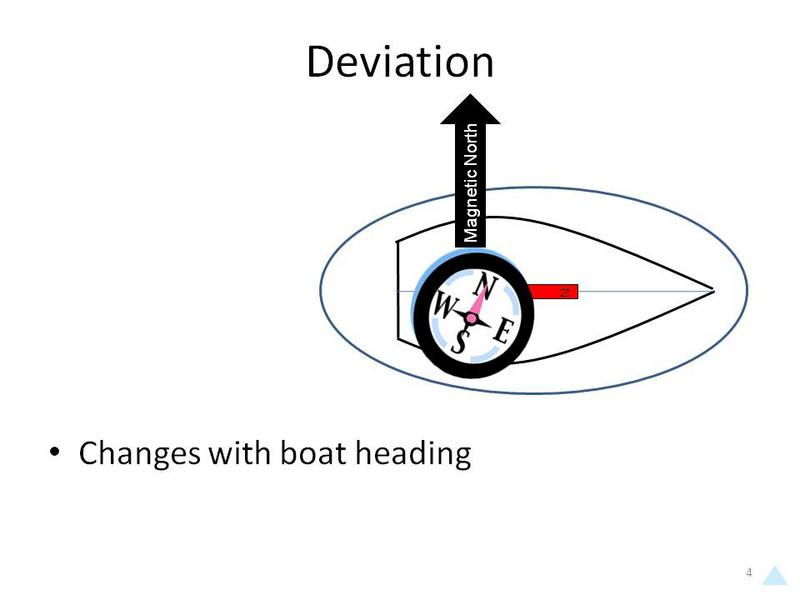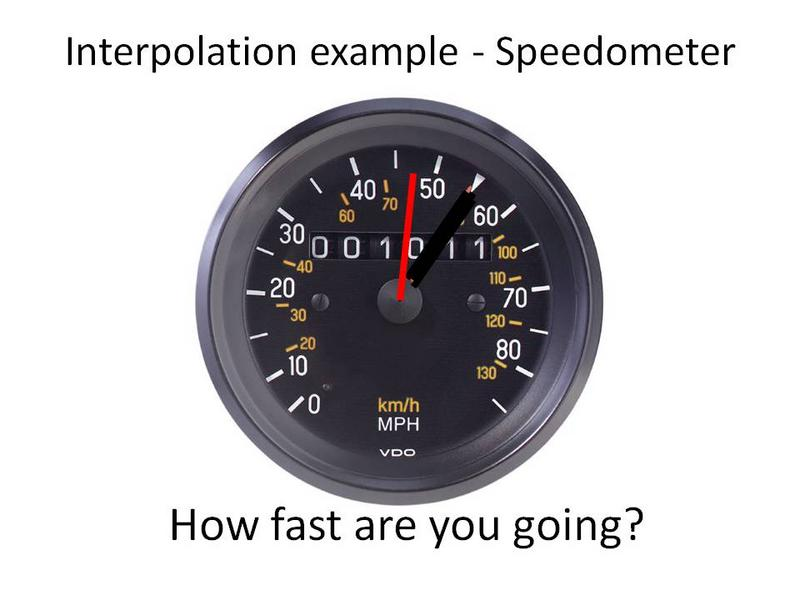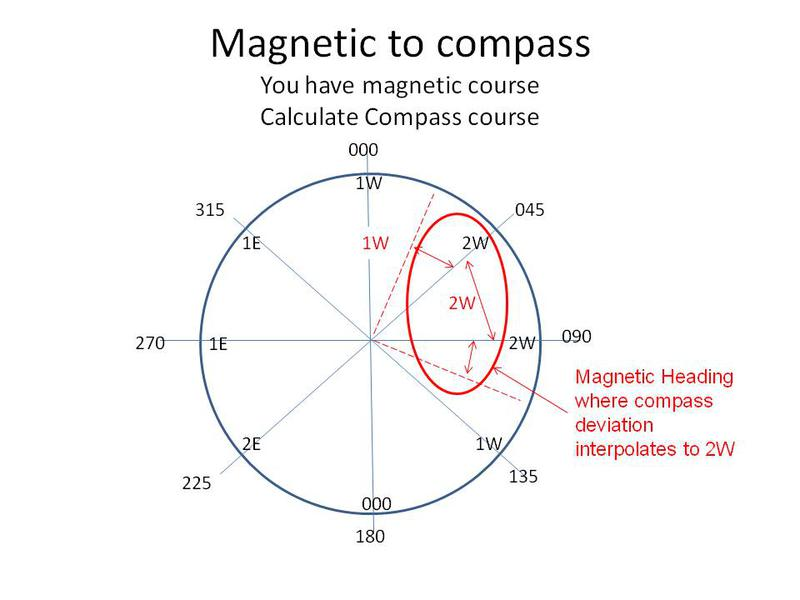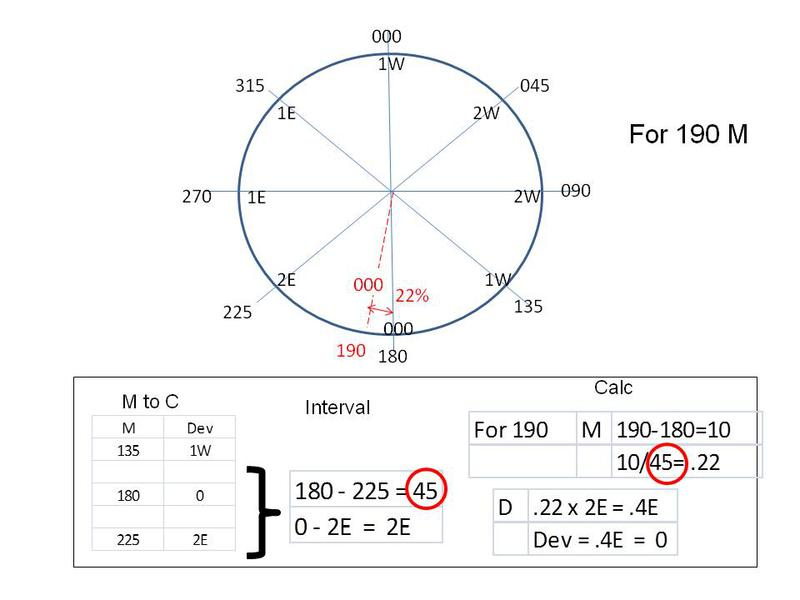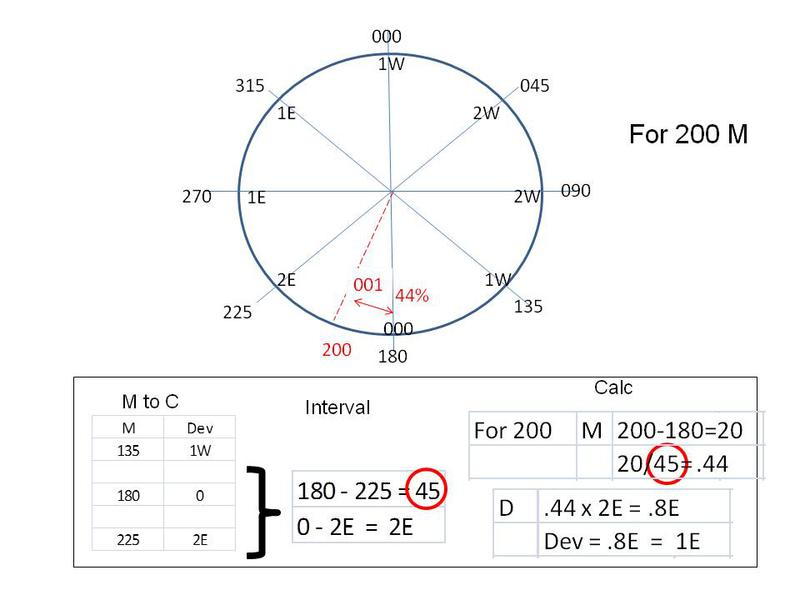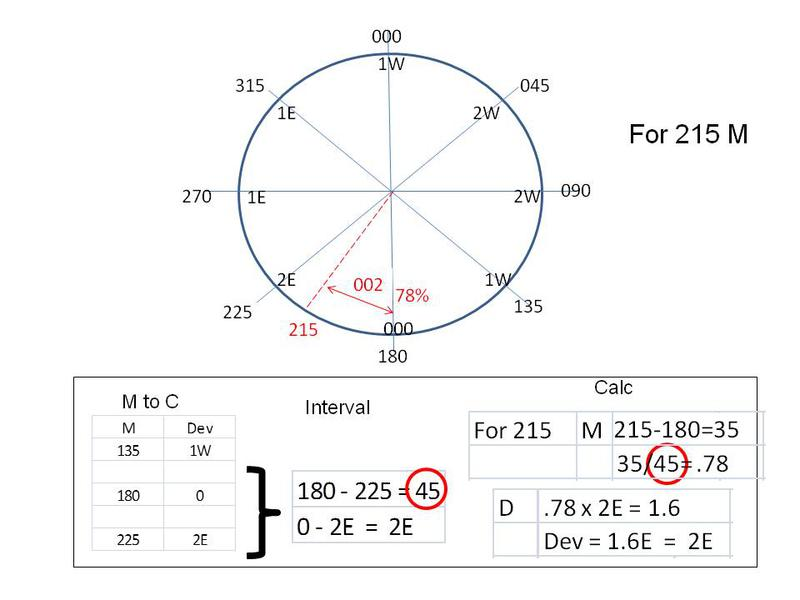Deviation depends on all the magnetic materials on your boat - engine block, metalic structures, electric fields, etc.

As your vessel changes direction, these materials on you boat "deflect" your compass in a different direction.

Each boat has a unique deviation table, depending on the magnetic materials onboard.
You interpolate by determining the "percentage" of your heading between two known deviation values.  In the first case below, to calculate the deviation at 190, you see that 190 is 22% (10/45) between 180 and 225.  Your deviation then is 22% between the deviation at 180 (which is 0) and the deviation at 225 (which is 2E).   22% of 2 is .44, which rounds to zero - so deviation in this case is zero.

In the second case below, deviation at 200 is 44% between deviation at 180 and 225, which calculates to .88 E and rounds to the nearest degree - 1E.

In the third example, you are 78% of the way to 225, so deviation calculates to 2E.

Don't forget to keep track of the direction of the deviation.  Going from 1E to 1W is a "gap" of 2 degrees of variation.

For instance, 335 is 44% of the way between 315 and 000.  The "gap" is 2 degrees.  44% of 2 degrees is .8, which rounds off to 1 degree.   Since you are moving from 1E (at 315) toward 1W (at 000), you subtract the 1 from 1E and get a deviation of zero.   Simple!